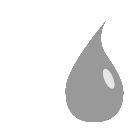# SmoothBilateral node¶This documentation is for version 2.0 of SmoothBilateral (net.sf.cimg.CImgBilateral).

## Description¶

Blur input stream by bilateral filtering.

Uses the ‘blur_bilateral’ function from the CImg library.

CImg is a free, open-source library distributed under the CeCILL-C (close to the GNU LGPL) or CeCILL (compatible with the GNU GPL) licenses. It can be used in commercial applications (see http://cimg.eu).

## Inputs¶

Input Description Optional
Source   No
Spatial Std Dev / sigma_s Double 10 Standard deviation of the spatial kernel (positional sigma), in pixel units (>=0). A reasonable value is 1/16 of the image dimension. Small values (1 pixel and below) will slow down filtering.
Value Std Dev / sigma_r Double 0.3 Standard deviation of the range kernel (color sigma), in intensity units (>=0). A reasonable value is 1/10 of the intensity range. In the context of denoising, Liu et al. (“Noise estimation from a single image”, CVPR2006) recommend a value of 1.95*sigma_n, where sigma_n is the local image noise. Small values (1/256 of the intensity range and below) will slow down filtering.
Iterations / iterations Integer 2 Number of iterations.
(Un)premult / premult Boolean Off Divide the image by the alpha channel before processing, and re-multiply it afterwards. Use if the input images are premultiplied.
Invert Mask / maskInvert Boolean Off When checked, the effect is fully applied where the mask is 0.
Mix / mix Double 1 Mix factor between the original and the transformed image.